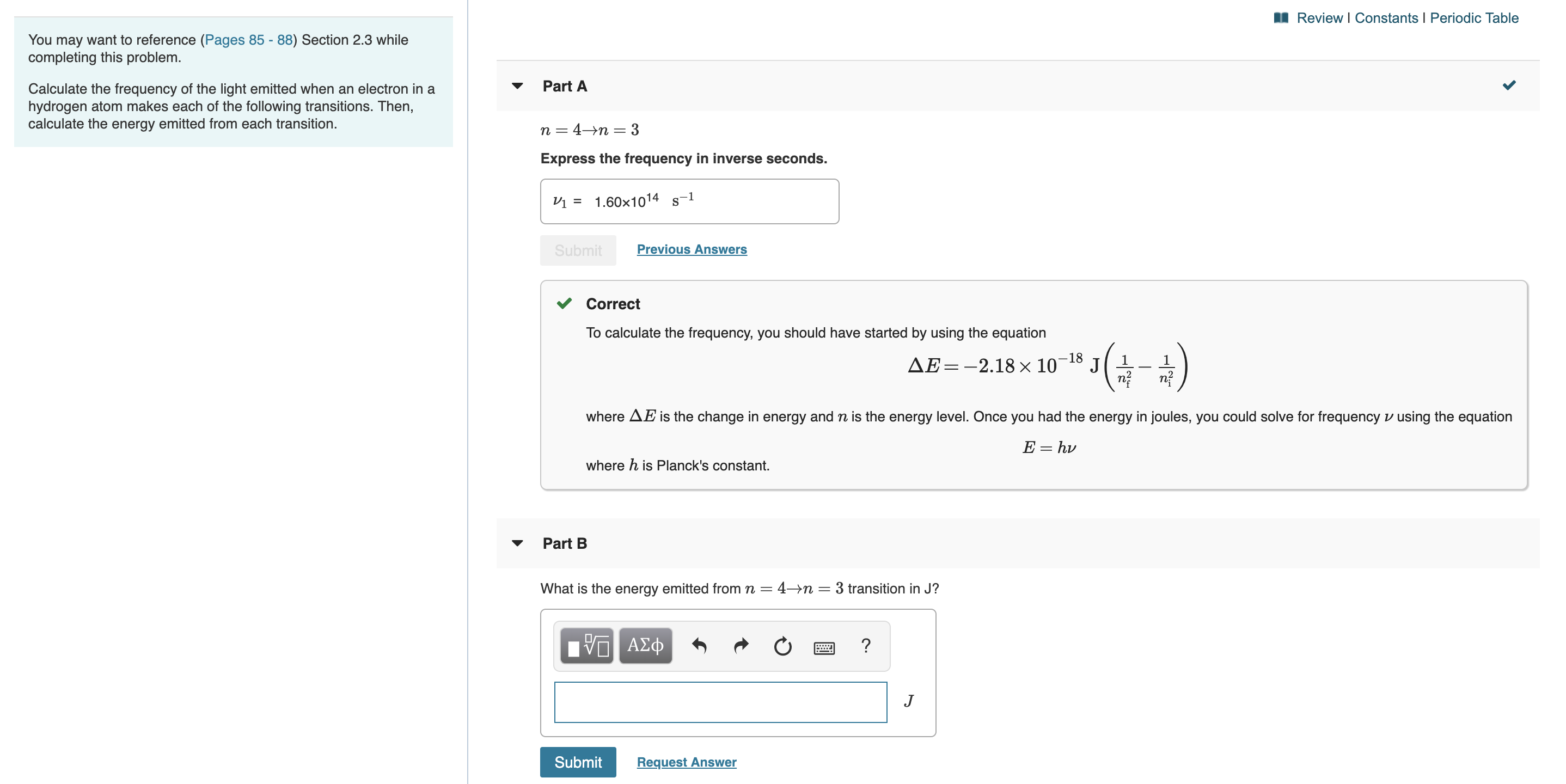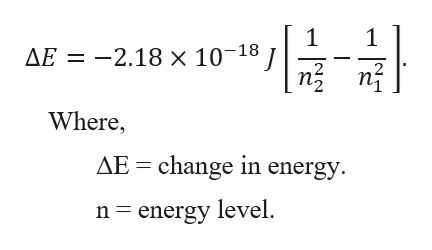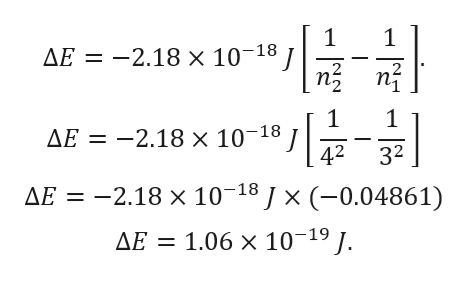Review I Constants I Periodic TableYou may want to reference (Pages 85 - 88) Section 2.3 whilecompleting this problem.Part ACalculate the frequency of the light emitted when an electron in ahydrogen atom makes each of the following transitions. Then,calculate the energy emitted from each transition.n 4-n 3Express the frequency in inverse seconds.1.60x1014 s-1Previous AnswersSubmitCorrectTo calculate the frequency, you should have started by using the equation-18J 11AE=-2.18x 10where AE is the change in energy and n is the energy level. Once you had the energy in joules, you could solve for frequency v using the equationE hvwhere h is Planck's constant.Part BWhat is the energy emitted from n = 4->n= 3 transition in J?V0 ΑΣφ?JSubmitRequest Answer

Questionhelp_outlineImage TranscriptioncloseReview I Constants I Periodic Table You may want to reference (Pages 85 - 88) Section 2.3 while completing this problem. Part A Calculate the frequency of the light emitted when an electron in a hydrogen atom makes each of the following transitions. Then, calculate the energy emitted from each transition. n 4-n 3 Express the frequency in inverse seconds. 1.60x1014 s-1 Previous Answers Submit Correct To calculate the frequency, you should have started by using the equation -18 J 1 1 AE=-2.18x 10 where AE is the change in energy and n is the energy level. Once you had the energy in joules, you could solve for frequency v using the equation E hv where h is Planck's constant. Part B What is the energy emitted from n = 4->n= 3 transition in J? V0 ΑΣφ ? J Submit Request Answer fullscreen
Step 1

The hydrogen atom is a single electron atom. The energy in a hydrogen atom depends on the energy of the electron. When the electron changes levels, it decreases energy and the atom emits photons. The photon is emitted with the electron moving from a higher energy level to a lower energy level. The energy of the photon is the exact energy that is lost by the electron moving to its lower energy level.help_outlineImage Transcriptionclose1 AE 2.18 x 10-18 1 n Where, AE change in energy n energy level fullscreen
Step 2

Given:

Higher energy level (n2) = 4.

Lower energy level (n1) =3.

Step 3

Calculation for chan...help_outlineImage Transcriptionclose1 AE = -2.18 x 10 18 n3 1 1 -2.18 x 10-18 1 AE 32 42 AE 2.18 x 10 18J x (-0.04861) AE 1.06 x 10-19/. fullscreen

Want to see the full answer?

See Solution

Want to see this answer and more?

Our solutions are written by experts, many with advanced degrees, and available 24/7

See Solution
Tagged in

Chemistry